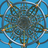首页   注册   登录# mathzhaoliang

V2EX 第 249225 号会员，加入于 2017-08-17 14:59:25 +08:00

19256mathzhaoliang 创建的所有主题提问技术话题好玩工作信息交易信息城市相关
 搞定了在 hexo 博客中运行 shader 程序的问题 分享创造  •  mathzhaoliang  •  14 小时 43 分钟前  •  最后回复来自 mathzhaoliang 3
 求助一个 Hexo 主题的 CSS 格式的修改 Hexo  •  mathzhaoliang  •  10 天前
 在 Linux 下怎样用 C 语言仅使用标准/系统库函数，获取物理 mac 地址 问与答  •  mathzhaoliang  •  255 天前  •  最后回复来自 ysc3839 2
 怎样监测一个程序是否正在运行 问与答  •  mathzhaoliang  •  258 天前  •  最后回复来自 xomix 11
 很讨厌 github 上的一种风气 GitHub  •  mathzhaoliang  •  261 天前  •  最后回复来自 Cukuyo 37
 上班划水，制作了两张分形图 分享创造  •  mathzhaoliang  •  265 天前  •  最后回复来自 caqiko 7
 给项目制作文档，哪种方式更好？ GitHub  •  mathzhaoliang  •  275 天前
 发现一个高产的 githuber 问与答  •  mathzhaoliang  •  299 天前  •  最后回复来自 hyyou2010 1
 pywonderland 项目的新作品集发布啦  1    分享创造  •  mathzhaoliang  •  300 天前  •  最后回复来自 Pudge1337 1
 求助一个 RSA 解密的问题 问与答  •  mathzhaoliang  •  305 天前  •  最后回复来自 Yanni0507 6
mathzhaoliang 最近回复了
 14 小时 43 分钟前
 14 小时 45 分钟前
@codehz

# 在 Hexo 中运行 shader 的步骤

## 下载 js 和 css 文件

## 开启可选加载


<% if (item.glsl) { %>
<script type="text/javascript" src="/glsl/glslEditor.js"></script>

<script type="text/javascript" src="/glsl/GlslCanvas.js"></script>

<script type="text/javascript" src="/glsl/GlslPost.js"></script>

<% } %>




(加在哪里不用我解释吧~)


glsl: true



<div align="center">
</div>


## 修改 mathjax 渲染器

glslEditor.css 的设置对 mathjax 数学公式的显示会有影响，应修改为 SVG 或者 HTML-CSS 渲染，所以需要修改 layout/_partial/plugin/mathjax.ejs 文件，在 MathJax.Hub.Config 语句的后面加上


MathJax.Hub.Queue(
["Typeset",MathJax.Hub,"HTMLCSS-output"],
["setRenderer",MathJax.Hub,"SVG"],
["Typeset",MathJax.Hub,"SVG-output"]
);


## 测试运行效果


<div class="codeAndCanvas" data="#ifdef GL_ES
precision mediump float;
#endif

uniform vec2 u_resolution;
uniform float u_time;
void main() {
vec2 pos = 256.0 * gl_FragCoord.xy / u_resolution.x + u_time;
vec3 col = vec3(0.0);
for (int i=0; i<6; i++) {
vec2 a = floor(pos);
vec2 b = fract(pos);
vec4 w = fract((sin(a.x * 7.0 + 31.0 * a.y + 0.01 * u_time) +
vec4(0.035, 0.01, 0.0, 0.7)) * 13.545317);
col += w.xyz * smoothstep(0.45, 0.55, w.w) *
sqrt(16.0 * b.x * b.y * (1.0 - b.x) * (1.0 - b.y));
pos /= 2.0;
col /= 2.0;
}
col = pow(2.5 * col, vec3(1.0, 1.0, 0.7));
gl_FragColor = vec4(col, 1.0);
}"></div>

<br/>

http://pywonderland.com/post/mobius-cn.html
 10 天前 回复了 magic3584 创建的主题 › 程序员 › 怎么刷 leetcode 最有效的了解数据结构和提升算法

 44 天前

 53 天前 回复了 yankqiang 创建的主题 › 分享创造 › 大家第一个项目，都是从网址导航开始？

https://neozhaoliang.github.io/